>### Master Math with 1:1 Online Classes. Learn from Experts!# Roman Numerals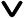• Introduction
• History
• How different is it from other systems?
• Symbols used in Roman Numerals
• Rules for writing Roman Numerals
• Roman Numerals Chart
• How to Write Roman numerals?
• Conversions between Two Systems
• Applications in Modern Times
• Solved examples
• Practice problems

## Introduction

In the numerical systems, Roman numerals stand as a fascinating and enigmatic method of representation. Roman numerals are a unique way of writing numbers that have been around for a long time. Even though we mostly use our regular numbers nowadays, Roman numerals have managed to persist through the ages.

## History of Roman Numerals

“The Romans found that using fingers gets tricky as the counting numbers go beyond ten.”

Roman numerals originated in ancient Rome with the Etruscans for the purpose of counting and performing other things. A piece of wood or a candlestick was used as a way of counting and recording the number of sheep. But as numbers grew larger for things like trading, marking dates, and counting pages in books, it became difficult to count on candles or wood sticks. So people used Roman numerals. For example, the numeral "7" represents the number seven. So, numerals are like codes that tell us the value of the number.

The system evolved over time and was used extensively throughout the Roman Empire. Unlike our regular numbers that depend on place value and powers of ten, Roman numerals are about adding and subtracting, which makes them special.

## How different is it from other systems?

The following Roman numbers hold a numeric value of Hindu-Arabic numbers. Mostly, these symbols are used in different arrangements for writing Roman numerals.## Symbols used in Roman Numerals

Romans in ancient times used different representations for conveying the idea of numbers. They used the English alphabet as a symbol to represent the numbers.

Not all alphabets are used in the Roman numeral system. Only Seven Roman symbols exist, and they're used to create thousands of different numbers. These symbols are Latin letters that stand for specific values.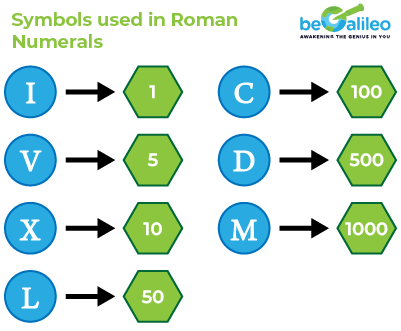Roman numerals are written as a combination of the seven letters.

## Rules for writing Roman Numerals

Rule 1: Usage of symbols:

• I, X, C, and M stand for numbers in multiples of 10, and can be used up to three times.
• V, L, and D represent numbers in multiples of 5, and can be used only once.

Rule 2: Use of Subtraction and addition of the seven letters.

If

I = 1

II = 1 + 1 = 2

III = 1 + 1 + 1 = 3

then ? = 4.

“FOUR SAME LETTERS CANNOT BE IN A ROW.”

So 4 is not IIII, but represented as five minus one(IV).

9 is not VIIII (5 + 1 + 1 +1 +1) but ten minus one(IX).

Hence, placement of the letters is important.

A number is subtracted if a letter denoting a smaller number appears before a letter denoting a larger number.For larger numbers, To avoid the repetition of certain symbols and enhance readability, Roman numerals incorporate subtractive notation.

The table shows the allowable combinations that can be used for subtraction.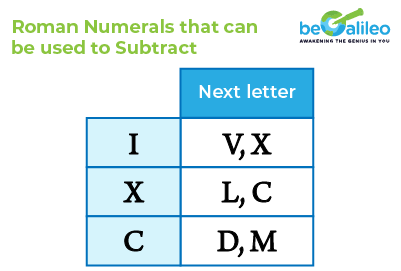What will happen if the number I comes after X , (ie) a smaller number after a larger number?

A number is added if a letter denoting a smaller number appears after a letter denoting a larger number.

XI = 10 + 1 = 11Add the values if the letter on the left has a value greater than or equal to the value of the letter next to it.

Rule 3: There is no zero in the Roman numerals system. The word NULLA is used to denote zero.

Rule 4: Number beyond 4M's

For the numbers that are greater than MMMM cannot be denoted using the regular rules of roman numerals.

We have to use the overbar on the letters (Vinculum)which denotes that it's a multiple of 1000.

4000 is not represented as MMMM but as 4 x 1000(\overline{IV}).

## Roman Numerals Chart

The Roman numeral chart is a valuable tool for converting between Roman numerals and regular numbers. The list below demonstrates how various numbers are written using Roman numerals.

Roman Numerals 1 to 20

The list of numerals from 1 to 20 represented in their respective Roman numeral translations is called Roman Numerals 1 to 20. Roman Numerals 1 to 20 will make it simple for students to learn how to convert numbers into roman numerals.

The list of roman numerals from 1 to 20 is provided in the table below.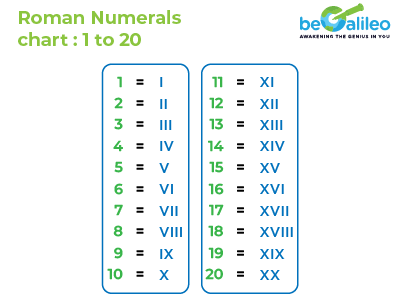To form numbers from 1 to 20:

• The numbers 1 to 10 follow the basic symbols and the rules for forming numbers.
• For 4, "IV" represents 5 - 1, subtracting 1 from 5.
• For 9, "IX" represents 10 - 1, subtracting 1 from 10.
• Numbers 11 to 20 are formed by combining the symbols for 10 (X) with the symbols for 1 to 10.

Roman Numerals 1 to 50

The set of numbers from 1 to 50 that are translated into Roman numerals is called Roman Numerals 1 to 50. Students can easily learn how to translate integers into Roman numerals by using Roman Numerals chart 1 to 50. Once kids understand Roman numerals from 1 to 50, they also learn how to create new numerals.

The list of roman numerals from 1 to 50 is provided in the table below.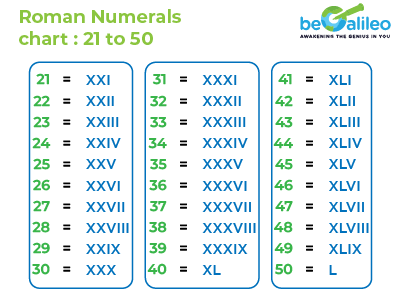To form numbers from 1 to 50:

• The numbers 1 to 10 follow the basic symbols and the rules for forming numbers.
• For 40, "XL" represents 50 - 10, subtracting 10 from 50.
• For numbers 11 to 20, "X" is added to the numbers 1 to 10.
• For numbers 21 to 39, "XX" is added to the numbers 1 to 19. For 10 (X) with the symbols for 1 to 10.
• For numbers 41 to 49, "XL" is added to the numbers 1 to 9.
• 50 is represented by "L".

Roman Numerals 1 to 100

The list of numerals from 1 to 100 represented in their respective Roman numeral translations is called Roman Numerals 1 to 100. Students can better understand the Roman numeral translations by being familiar with the Roman Numbers 1 to 100. Once kids understand Roman numbering from 1 to 100, they also learn how to create new numerals.

The list of roman numerals from 1 to 100 is provided in the table below.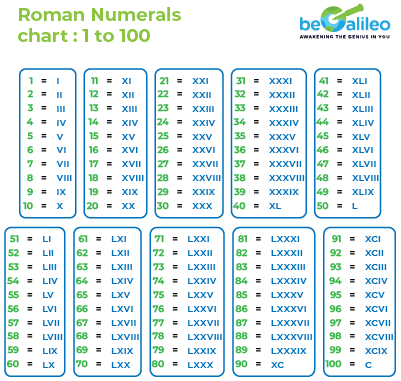Roman Numerals 101 to 200

The numbers from 101 to 200 shown as Roman numerals are called "Roman Numerals 101 to 200." Students can get a better grasp of Roman numerals by knowing these numbers. When kids know Roman numbers from 101 to 200, they can also figure out how to make new numerals.

The list of roman numerals from 101 to 200 is provided in the table below.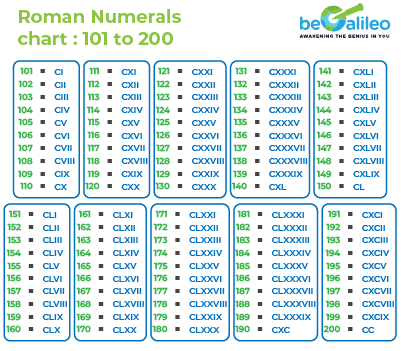Roman Numerals 201 to 300

Roman Numerals 201 to 300 is the collection of numbers from 201 to 300 shown in their corresponding Roman numeral forms. Familiarity with Roman Numbers 201 to 300 can enhance students' comprehension of Roman numeral translations. As children grasp Roman numbering between 201 and 300, they also acquire the skill of forming new numerals.

The list of roman numerals from 201 to 300 is provided in the table below.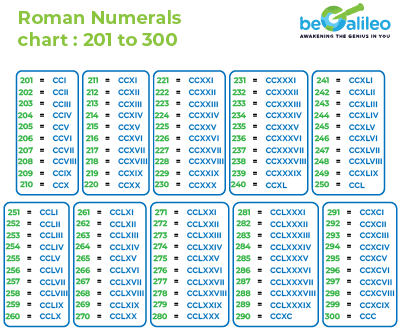Roman Numerals 301 to 400

Roman Numerals 301 to 400 refers to the list of numbers from 301 to 400 and their corresponding roman numeral representations. Knowing the Roman Numbers 301 to 400 will help students comprehend the Roman numeral translations. Kids learn how to make new digits if they comprehend Roman numbering from 301 to 400.

The list of roman numerals from 301 to 400 is provided in the table below.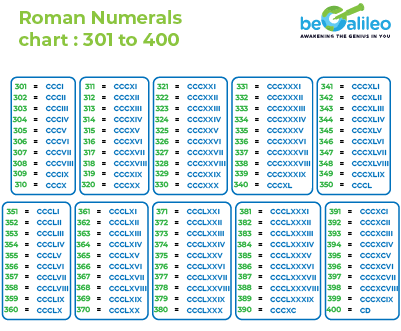Roman Numerals 401 to 500

The numerals from 401 to 500, each converted into their corresponding Roman numeral, make up the group known as Roman Numerals 401 to 500. Students' understanding of Roman numeral concepts is made easier by becoming familiar with Roman Numbers 401 to 500. Children who have a good knowledge of Roman numbers from 401 to 500 are able to make unique numerals.

The list of roman numerals from 401 to 500 is provided in the table below.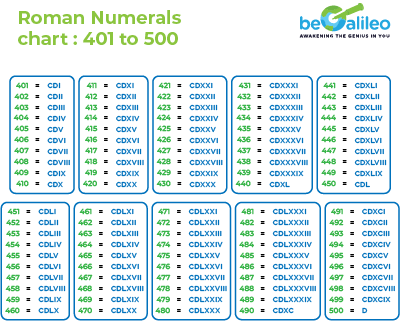Roman Numerals 501 to 1000

The compilation known as Roman Numerals 501 to 1000 consists of numbers spanning from 501 to 1000, each uniquely symbolized by a Roman numeral. Students can enhance their understanding of Roman numeral meanings by becoming acquainted with Roman Numbers 501 to 1000. The capacity to formulate new numerals is a skill that children cultivate as they explore Roman numbering within the range of 501 to 1000.

The list of roman numerals from 501 to 1000 is provided in the table below.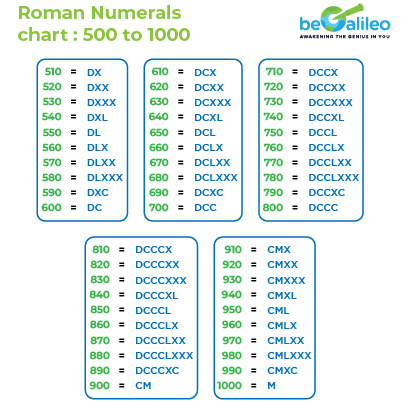## How to Write Roman Numerals?

To write Roman numerals, follow these steps:

1. Start from the largest symbol that fits within the number.
2. Write that symbol the required number of times according to the value you want to represent.
3. If a smaller symbol should come before a larger one, place the smaller symbol in front of the larger one to subtract its value.
4. Continue this process until you've represented the entire number.

Remember the rules about repetition and subtraction for certain symbols, as well as the use of the bar line to multiply by 1000. This way, you can create accurate Roman numeral representations.

## Conversions between Two Systems

Converting numbers between decimal (our standard number system) and Roman numeral systems involves translating the numeric value from one system to the other. Let's explore how to perform conversions in both directions.## Related Math Games

### Decimal to Roman Numerals:

1. Identify Place Values: In the decimal system, numbers are grouped by place values (ones, tens, hundreds, etc.). Identify the place values of the number you want to convert.
2. Break Down the Number: Break down the decimal number into its individual place values (e.g., 364 = 300 + 60 + 4).
3. Find the Corresponding Roman Numerals:For each place value, find the corresponding Roman numeral using the basic symbols (I, V, X, L, C, D, M) and their values.
4. Combine Roman Numerals: Write the Roman numeral equivalents of each place value together to form the final Roman numeral representation.

Example: Convert 364 to Roman Numerals:

1. Breakdown: 364 = 300 + 60 + 4
2. Roman Numerals: 300 = CCC, 60 = LX, 4 = IV
3. Combine: CCC + LX + IV = CCCLXIV

### Roman Numerals to Decimal:

1. Identify Symbols: Look at the Roman numeral and identify its symbols (I, V, X, L, C, D, M).
2. Combine Values: Combine the values of the symbols from left to right. If a smaller symbol appears before a larger one, subtract its value. If a smaller symbol appears after a larger one, add its value.
3. Sum Up: Sum up the values of the symbols to obtain the decimal equivalent.

Example: Convert CCCLXIV to Decimal:

1. Symbols: CCCLXIV (CCC + LX + IV)
2. Values: CCC = 300, LX = 60, IV = 4
3. Sum: 300 + 60 + 4 = 364

## Applications in Modern Times

While the use of Roman numerals has waned significantly in everyday calculations, they persist in various aspects of our culture:

Clocks and Watches: Roman numerals are commonly used on clock faces to give them a classic and elegant appearance.Page Numbering: You might find Roman numerals used in the front matter of books, indicating sections like prefaces, introductions, and forewords.

Numbering Chapters and Sections: Some formal documents and academic papers use Roman numerals to label different sections.

Movie and TV Show Titles: Roman numerals are often used in the titles of movies, sequels, and TV shows to give them a unique and timeless feel.

Title of games: Title of each year Olympics or leagues like Super bowl etc use roman numerals in their titles.

## Solved Examples

### Q1. What is the value of the Roman numeral XLVIII?

1. a) 68
2. b) 48
3. c) 108
4. d) 98

Solution: Correct answer is b) 48.
We know that,
I = 1, x is 10, L is 50 and V is 5
X = 10 is less than L = 50, So subtract the values.
XL = 50 - 10 = 40
V = 5 is greater than I = 1, so add the values.
VIII = 5 + 1 + 1 + 1 = 8.
Therefore, XLVIII = 48.

### Q2. Ben wants to go to see his favorite show on the TV. The advertisement says the show starts at 9. Ben says when the shorthand of the clock points at the Roman numeral XI, then the show starts. Is he correct?

1. a) Yes
2. b) No

Solution: The correct answer is b) No.
We know that,
x = 10, and I = 1
X = 10 is greater than I = 1, so add the values.
XI = 10 + 1 = 11
Thus, the time is 11 o'clock and the show starts
at 9 o’ clock.
Hence, Ben is incorrect.

### Q3. Sam and Diya convert 90 into Roman numerals. Sam says 90 = LXXXX, and Diya says 90 = XC. Who is correct?

1. a) Sam
2. b) Sara
3. c) Both of them
4. d) None of them

Solution: The correct answer is b) Diya.
We know that,
I, X, C, and M stand for numbers in multiples of 10, and can be used up to three times.
Sara calculated as shown, Which is not valid.
90 =50+10+10+10+10
=L+X+X+X+X
=LXXXX
Diya calculator as shown,
90=100-10
=C-X
=XC
Hence, Diya is correct.

### Q4. Choose the Roman numeral for the number 719.

1. a) DCCXIX
2. b) MCCXIX
3. c) DCXIX
4. d) DCCCXIX

Solution: The correct answer is a) DCCXIX.
Step1: Express the number as a sum.
719 = 700+10 + 9
We know that, D = 500, C = 100, X = 10 and IX = (10 - 1) = 9
Then, 700 = DCC, X = 10, and 9 = IX
719 = 700+10 + 9 = DCC+ X + IX
Thus, 719= DCCXIX.

### Q5. What does the Roman number \overline{D} represents?

1. a) 500
2. b) 50000
3. c) 5000
4. d) 500000

Solution: The correct answer is d) 500000.
The Roman number D has a value equivalent to 500.
Rule: The bar on the alphabet represents the "value multiplied by 1000".
Thus, D has a value equivalent to 500 x 1000= 500000.
Hence \overline{D} = 500000

## FAQs - Roman Numerals

Roman numerals are a system of writing numbers using specific letters from the Latin alphabet. They were used by ancient Romans and are still occasionally used today.

Roman numerals are read by adding the values of the letters from left to right. If a smaller value appears before a larger value, you subtract the smaller value.

The basic Roman numeral symbols are I (1), V (5), X (10), L (50), C (100), D (500), and M (1000).

Larger numbers are formed by combining these symbols and following rules for repetition and subtraction. For example, 4 is represented as IV (5 - 1), and 9 is represented as IX (10 - 1).

There is no defined limit, but Roman numerals can become lengthy and cumbersome for very large numbers.

Roman numerals are often seen on clock faces, in movie titles, book chapters, building cornerstones, and for indicating the order of monarchs and popes.

Roman numerals are not as efficient as our modern decimal system for arithmetic calculations. They are more suited for symbolic and decorative purposes.

To convert Roman numerals to regular numbers, add the values of the symbols. To convert regular numbers to Roman numerals, find the largest symbols that fit within the number and combine them.

Yes, some key rules include not using more than three of the same symbol in a row (except for M), using subtractive notation for 4, 9, 40, 90, etc., and using certain symbols only once (V, L, D).

Roman numerals are often taught in educational settings as part of historical and cultural lessons. However, they are not commonly used for arithmetic calculations.

You can practice by converting numbers to and from Roman numerals, solving exercises, and using online resources and quizzes dedicated to Roman numerals.